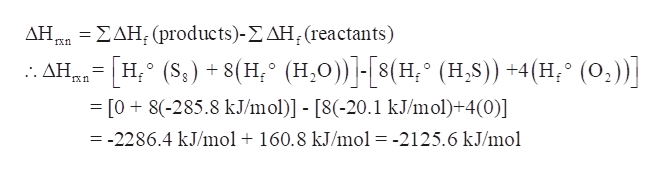# Using the table of standard formation enthalpies, calculate the reaction enthalpy of this reaction under standard conditions: 8H2S+4O2-->S8+8H2O

Question
64 views
Using the table of standard formation enthalpies, calculate the reaction enthalpy of this reaction under standard conditions:

8H2S+4O2-->S8+8H2O
check_circle

Step 1

Using the table of standard formation enthalpies, the reaction enthalpy of this reaction under standard conditions is to be determined:

8H2S+4O2→S8+8H2O

Step 2

The enthalpy of reaction is the change in enthalpy (total heat content) of a reaction that occurs at constant pressure.

Mathematically, the enthalpy of a reaction (ΔHrxn) is the difference between the total enthalpy of formation of the products and the total enthalpy of formation of reactants.

Step 3

Now, for the given reaction, standard formation enthalpies are-

Hf° (H2S) = -20.1 kJ/mol

Hf° (O2) = 0 kJ/mol

Hf° (S8) = 0 kJ/mol

Hf° (H2O) = -285.8

Therefore,

ΔHrxn = [Hf° (S8) + 8(Hf°...help_outlineImage TranscriptioncloseΔΗ ΣΔΗ, (products) - Σ ΔΗ, (reactants) - [Η (5,) + (H * (H,Ο)]-[s(H, (H.S)) +4(Η, * (0,)] = [08(-285.8 kJ/mol)] - [8(-20.1 kJ/mol)+4(0)] Ε Χη =-2286.4 kJ/mol 160.8 kJ/mol = -2125.6 kJ/mol fullscreen

### Want to see the full answer?

See Solution

#### Want to see this answer and more?

Solutions are written by subject experts who are available 24/7. Questions are typically answered within 1 hour.*

See Solution
*Response times may vary by subject and question.
Tagged in

### Physical Chemistry